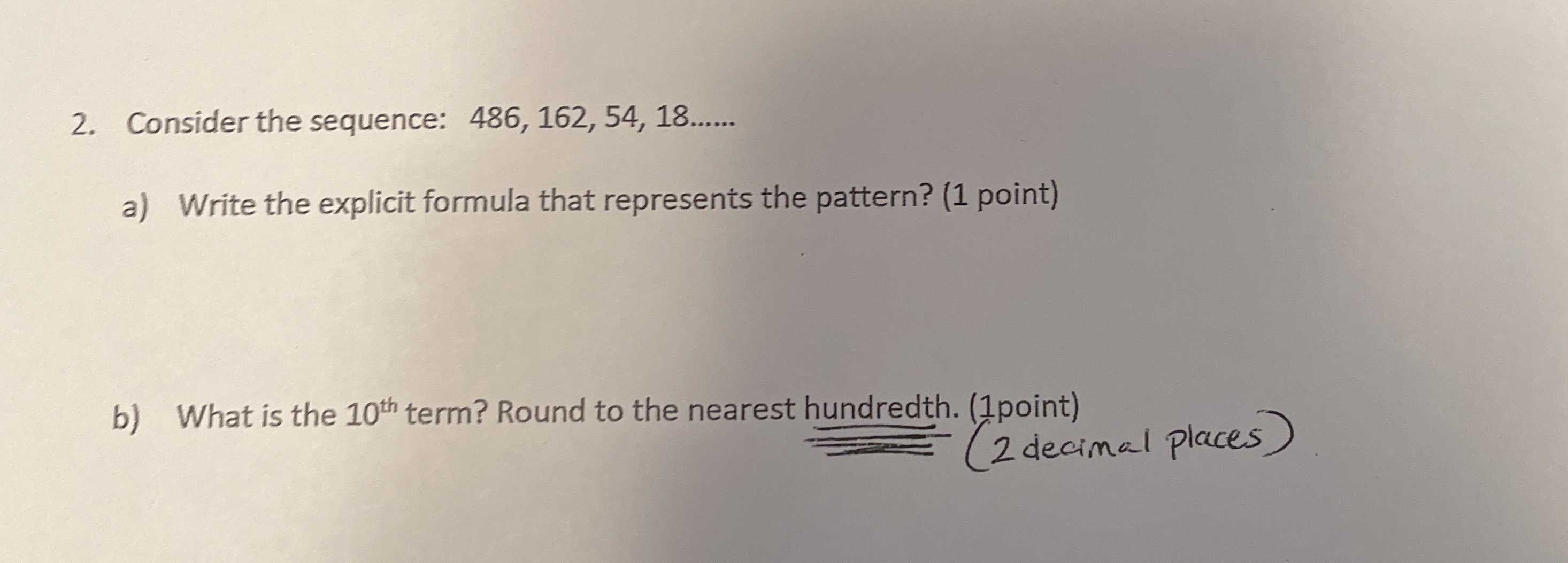### ¿Todavía tienes preguntas de matemáticas?

Pregunte a nuestros tutores expertos
Algebra
Pregunta2. Consider the sequence: $$486,162,54,18 \ldots \ldots$$

a) Write the explicit formula that represents the pattern? (1 point)

b) What is the $$10 ^ {$$ th $$}$$ term? Round to the nearest hundredth. (1point) (2 decimal places)

486×($$\frac{1}{3}$$)$$^{n}$$